Predator prey system

(diff) ← Older revision | Latest revision (diff) | Newer revision → (diff)

The predator prey problem refers to an ecological system in which we have two species, one of which feeds on the other. This type of system has been studied for decades and is known to exhibit very interesting dynamics. The right figure below shows a historical record taken over 50 years in the population of lynxes versus hares.

As can been seen from the graph, the annual records of the populations of each species are oscillatory in nature.

Modeling

Discrete time model

A simple model for this situation can be constructed using a discrete time model by keeping track of the rate of births and deaths of each species. Letting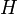represent the population of hares and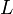represent the population of lynxes, we can describe the state in terms of the populations at discrete periods of time. Letting \$k\$ be the discrete time index (e.g., the day number), we can write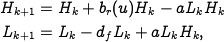where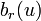is the hare birth rate per unit period and as a function of the food supply,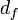is the lynx death rate, and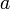is the interaction term. The interaction term models both the rate at which lynxes eat hares and the rate at which lynxes are produced by eating hares. This model makes many simplifying assumptions -- such as the fact that hares never die of old age or causes other than being eaten -- but it often is sufficient to answer basic questions about the system.